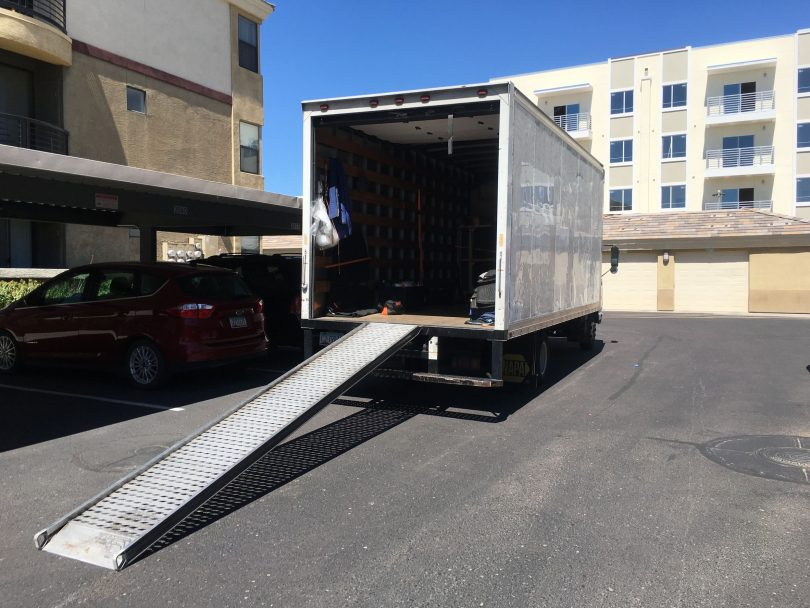Compare Movers and Prices
Moving Cost

# How to Calculate Linear Feet the Right WayThere are a lot of factors that go into calculating how much your move will cost. To ensure that you go in with a complete understanding of what your movers will be using to determine your estimate and final cost, we’ll explain how to calculate linear feet the right way — at least, the right way for the moving industry.

## What is a linear foot?

A linear foot, also referred to as a “lineal foot”, is 12 inches or one foot. The word “linear” just means the measurement refers to a straight line.

## What sets it apart from other measurements?

Many methods of measurement, like cubic square feet and cubic feet, account for width and height as well as linear distance. If you are determining how much space you need in a moving truck or how much of some building materials you will need for a project, the width and height will be fixed. All you need to determine is the linear, or straight-line, length.

## In what instances would you need to know, and how do calculate a linear foot?

Home improvement projects like building decks, fencing, or bookshelves will generally utilize materials with fixed widths, meaning you only need to know how many feet of each material you will need. For example: if you are using 2×4” boards, you may need 8 linear feet of board. For moving, many companies determine the cost of your move by how much linear feet your cargo takes up in the truck. To measure linear feet, measure the length in inches, then divide by 12 to determine linear feet. No complicated calculations required, as when you’re measuring square footage!

## Why is this important to know for moving?

Many moving companies use linear foot measurements to determine the cost of a given move. Using markers for the linear feet inside a trailer, your initial quote will allow for a certain number of linear feet capacity. When you are at the stage of trying to estimate the cost of your move, it is important to know what this distance means so that you can best estimate how much cargo you will need to be loaded and how much that will likely cost. Your quote will likely include a “per-foot adjustment rate” in case you need less or more space on the truck. Of course, learning how pack and load a moving truck well (side to side as well as utilization of space) can make a big difference in efficiently using your allotted space on the truck and therefore cost.

Tip: if you’re wondering how to convert square feet to linear feet, there is no direct translation since square feet accounts for more factors. You’ll just need to measure the linear length.

With highly skilled and professional local moving companies, you won’t need to worry too much about making all sorts of measurements. They’ll help you understand how to best transport your cargo in a cost-effective manner!